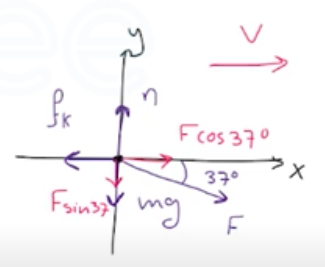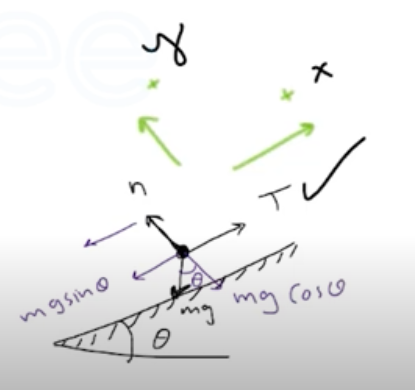Need Help?

Subscribe to Physics 1

###### \${selected_topic_name}
• Notes

$\begin{array}{c}{\text { A. } 22 \text { rifle bullet, traveling at } 350 \mathrm{m} / \mathrm{s} \text { , strikes a large }} \\ {\text { tree, which it penetrates to a depth of } 0.130 \mathrm{m} \text { . The mass of the }} \\ {\text { bullet is } 1.80 \mathrm{g} \text { . Assume a constant retarding force. (a) How much }} \\ {\text { time is required for the bullet to stop?( b) What force, in newtons, }} \\ {\text { does the tree exert on the bullet? }}\end{array}$

$V_{0 x}=350 \mathrm{m/s}$

$V_{x}=0$

$x-x_{0}=0.13 \mathrm{m}$

$\left(x- x_{0}\right)=\left(\frac{v_{0 x}+v_{x}}{2}\right) t$

$t=\frac{2\left(x-x_{0}\right)}{V_{0 x}+V_{x}}=\frac{2(0.13)}{350}$

$=7.43 * 10^{-4} \mathrm{S}$

$\sum F_{x}=m a_ x$

$-F=ma_x \Rightarrow F=-ma_x$

$=-\left(1.8 * 10^{-3}\right)\left(a_{x}\right)$

$V_{x}^{2}=V_{0x}^{2}-2a_ x\left(x-x_{0}\right) \rightarrow a_{x}=\frac{\left(V_{x}^{2}-V_{0 x}^{2}\right)}{2\left(x+x_0\right)}=-4.71 \times 10^{5}m/s^2$

$F_{x}=848 N$

$\begin{array}{l}{\text { A chair of mass } 12.0 \mathrm{kg} \text { is sitting on the horizontal floor; }} \\ {\text { the floor is not frictionless. You push on the chair with a force }} \\ {F=40.0 \mathrm{N} \text { that is directed at an angle of } 37.0^{\circ} \text { below the horizon- }} \\ {\text { tal and the chair slides along the floor. (a) Draw a clearly labeled }} \\ {\text { free-body diagram for the chair. (b) Use your diagram and Newton's }} \\ {\text { laws to calculate the normal force that the floor exerts on the chair. }}\end{array}$$a _y=Zero$

$\sum F_{y}=m g_{y}$

$\sum F_ y=0 \longrightarrow$

$n-m g-F \sin 37=0$

$n=m g+F \sin 37$

$n=(12)(9.8)+(40) \sin 37=142 \mathrm{N}$

$∴ n=142 N$

$\begin{array}{l}{\text { A skier of mass } 65.0 \mathrm{kg} \text { is pulled up a snow-covered slope }} \\ {\text { at constant speed by a tow rope that is parallel to the ground. The }} \\ {\text { ground slopes upward at a constant angle of } 26.0^{\circ} \text { above the hori- }} \\ {\text { zontal, and you can ignore friction. (a) Draw a clearly labeled free- }} \\ {\text { body diagram for the skier. (b) Calculate the tension in the tow rope. }}\end{array}$$\sum F_{x}=ma_{x}$

$T-m g \sin \theta=0$

$T=m g \sin \theta$

$=(65)(9.8) \sin 26^{\circ}$

$=279 N$